# wrepsher

•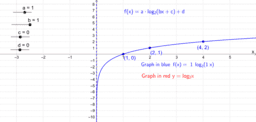### Graphs of Logarithmic Functions base > 1

Activity

wrepsher

•### Graphs of Logarithmic Functions

Activity

wrepsher

•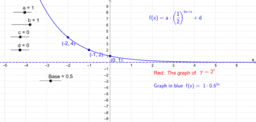### Graphs of Exponential Functions

Activity

wrepsher

•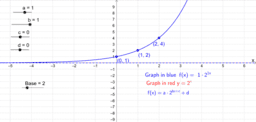### Graphs of Exponential Functions

Activity

wrepsher

•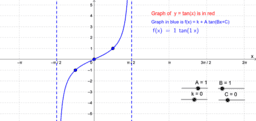### Tangent Graphs: y = k + A tan (Bx + C)

Activity

wrepsher

•### Sine Graphs: y = c + a sin [b(x – d)]

Activity

wrepsher

•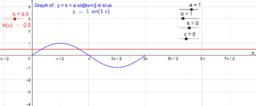### Solving Trigonometric Equations Graphically

Activity

wrepsher

•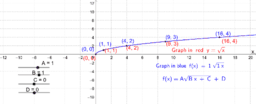### Transformations of the Square Root Function

Activity

wrepsher

•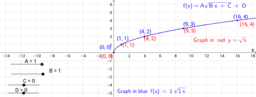### Rigid Transformations of Square Root Function

Activity

wrepsher

•### Graph Transformations of the Square Root Function

Activity

wrepsher

•### Logarithmic Functions 0 < Base < 1

Activity

wrepsher

•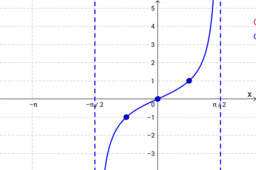### Tangent Graphs: y = c + a tan [b(x – d)]

Activity

wrepsher

•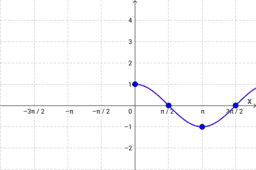### Cosine Graphs: y = c + a cos [b(x – d)]

Activity

wrepsher

•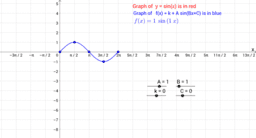### Sine Graphs: y = k + A sin(Bx + C)

Activity

wrepsher

•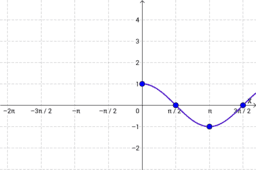Activity

wrepsher